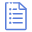# 高考复习-向量的加法和减法运算 课件（共19张PPT）资源简介

(共19张PPT)

1、向量的加法：
(1)、定义：求两个向量和的运算叫向量的加法。
(2)、图示：
b
a
O
a
a
a
a
a
a
a
a
b
b
b
b
b
b
b

B
b
a
A
(3)、作法
a+b

a
b

b
a
a
a
a
a
a
A
B
b
b
b
C
a
b
a
a
a
a
a
a
A
B
b
b
b
b
b
C

O

A
B
（1）
（2）
（3）
（4）

（4）平行四边形法则
b
a
A
a
a
a
a
a
a
a
a
b
b
b
B
b
a
D
a
C
b
a+b

（3）则以点A为起点的对角线AC＝a+b
（1）
（2）

1、向量的加法：
2、向量的加法的几何作法：
3、向量的加法的符号运算以及运算率：
a
b
a+b
a
a+b
b

1、向量的减法：求两个向量差的运算（差仍为向量）
2、向量的减法的几何作法：

a
b
a-b
－b
a+b

a-b

A
B
C
B
C
A

（1）
（2）
（3）
（4）

A
B
C
D资源预览